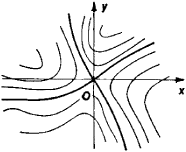Also found in: Wikipedia.

(geology)
(mathematics)
A point where all the first partial derivatives of a function vanish but which is not a local maximum or minimum.
For a matrix of real numbers, an element that is both the smallest element of its row and the largest element of its column, or vice versa.
For a two-person, zero-sum game, an element of the payoff matrix that is the smallest element of its row and the largest element of its column, so that the corresponding strategies are optimal for each player, given the strategy chosen by the other player.
McGraw-Hill Dictionary of Scientific & Technical Terms, 6E, Copyright © 2003 by The McGraw-Hill Companies, Inc.
The following article is from The Great Soviet Encyclopedia (1979). It might be outdated or ideologically biased.

a critical point of a first-order differential equation. In a neighborhood of a saddle point, four half-line integralFigure 1

curves enter the critical point. Between the four curves there are four regions, each of which contains a family of integral curves resembling hyperbolas (see Figure l). The pattern of integral curves in a neighborhood of a saddle point is reminiscent of the contour lines of a hyperbolic paraboloid, which has the shape of a saddle—hence the name of the critical point.

References in periodicals archive ?
Here, there is notion of a critical saddle point of 2%.
For bare cylinder case, two focus points (F1, F2) emerge in the close region of cylinder and a saddle point S occurs at the location which is considered at the end of vortex formation.
Stable Points DetJ TrJ Local Stability A(0, 0) + - ESS 5(0, 1) - Uncertainty Saddle point C(1, 0) + + Unstable 0(1, 1) - Uncertainty Saddle point Table 3: Local stability analysis of the evolution system.
According to the Karuch-Kuhn-Tucker conditions, a point of equilibrium for the A interaction is a saddle point for the win formula F , The meaning of this result, namely of the term 'saddle point' is revealed by the answer to the question: 'What does an optimum means of expression signify in the case of both initiators of interaction [DELTA]?'.
They cover linear optical response and Raman spectroscopy, nonlinear optical properties, terahertz response, saddle point spectroscopy, and device application.
In fact, such theorem considers a diffeomorphism [phi] possessing a transversal homoclinic point q to a hyperbolic saddle point p.
The saddle point [S.sub.*] corresponds to the point where the derivative of the phase function with respect to the t variable in the integrand in Equation (13) equals to zero.
Around a saddle point [z.sub.0], where f'([z.sup.0]) = 0, there are two constant-phase (steepest) contours, on which Im f(z) is constant, passing through [z.sub.0] if f"([z.sub.0]) [not equal to] 0.
However, it is a saddle point under the following conditions:
The saddle point method is used to determine the solution with zero group velocity.
For standard definitions of attracting fixed point, saddle point, stable manifold, and related notions see .

Site: Follow: Share:
Open / Close